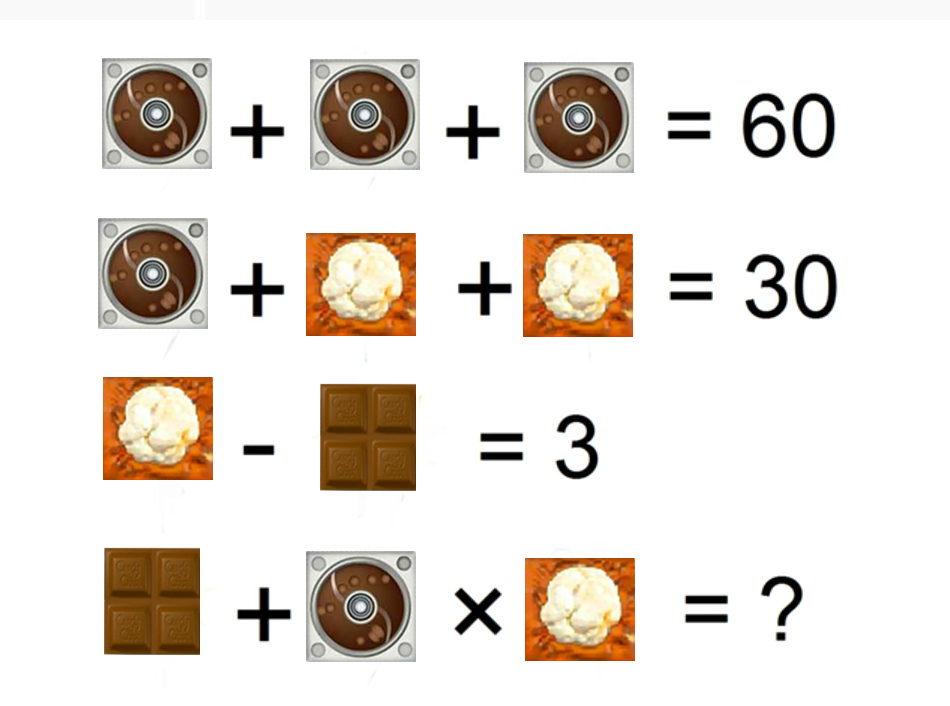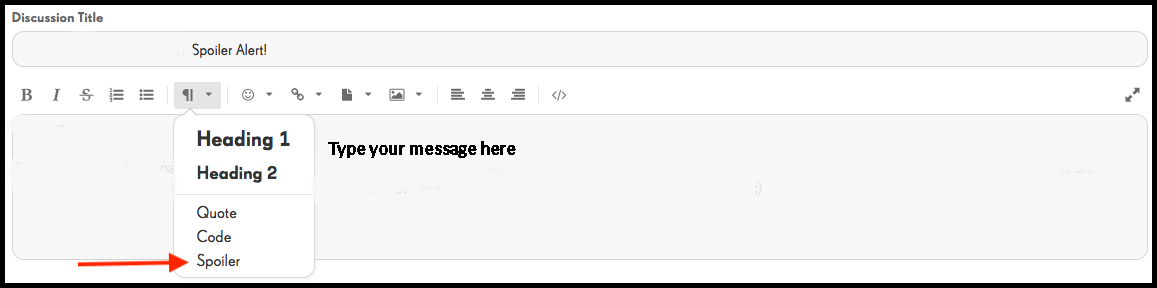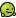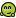# A different type of math practice

edited October 31

This is not a contest.  It’s just some fun time to get you used to the contests that we have in the community.
Below you will find a different type of match challenge.   Do the math with our Saga blockers to determine what the answer will be.You can practice doing the spoiler on this challenge.  Follow the arrow to use the spoiler.**update**

The correct answer to this is 110.Prev1

• edited October 23• And my answer is 630.
🎄❄🎄⚜️Contest Area🎀

Which is probably wrong😬😢

• Oops sorry by mistake I said 630 but correct answer is 110.
Because
2+20=22×5=110.
I know this is my second post and you chose my first post which is wrong.
🎄❄🎄⚜️Contest Area🎀

• edited October 26
Hi @Elsa , here is my answer I believe for this one2+20×5 = 110

Also @satnam , you are allowed to change your answers during a contest like this up until the contest date is over.However, please be sure to use the "spoiler" tag for your answers as per the rules of the contest which can be found here as @Elsa showed:Looking forward to seeing you guys in our other contests!• edited October 26
2+20×5=110

• So I guess I am probably the only one who got it wrong 😂
• 110

## Beginner's Corner

Check it out! It's not just for beginners of the game. Are you new to the Community? Click on the links below and check it out!• 2+20×5=102 multiple first then add
• 102It's going to be Friend-Tastic ❄️
Find out more here!
• edited October 30

2 (Chocolate Value) + 20 (Chocolate Spawner Value) x 5 (Popcorn Value) = 110 (Total)

Hi @Elsa

Are we following the PEMDAS (Please Excuse My Dear Aunt Sally) or the Order Of Operation (Parentheses, Exponents, Multiplication and Division, and Addition and Subtraction)? If we are, the answer will be different.

Using PEMDAS

20 (Chocolate Spawner Value) x 5 (Popcorn Value) + 2 (Chocolate Value) = 102 (Total)

Thanks!Life is a circle of happiness, sadness, hard times and good times.
If you are going through hard times, have faith that good times are on the way.

From Star Wars Episode IX Teaser. (Converted from MP4 to GIF Format by: mercerik)

• edited November 8
I was not happy with my first answer. Correction shown below !!!!

= 102

In mathematics and computer programming, the order of operations (or operator precedence) is a collection of rules that reflect conventions about which procedures to perform first in order to evaluate a given mathematical expression.

For example, in mathematics and most computer languages, multiplication is granted a higher precedence than addition, and it has been this way since the introduction of modern algebraic notation. Thus, the expression 2 + 3 × 4 is interpreted to have the value 2 + (3 × 4) = 14, not (2 + 3) × 4 = 20. With the introduction of exponents in the 16th and 17th centuries, they were given precedence over both addition and multiplication and could be placed only as a superscript to the right of their base. Thus 3 + 52 = 28 and 3 × 52 = 75.

#### Howdy, Stranger!

It looks like you're new here. If you want to get involved, click one of these buttons!